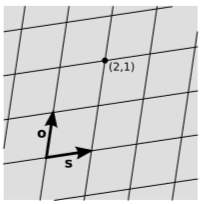# 1.2: Minkowski Coordinates

$$\newcommand{\vecs}{\overset { \rightharpoonup} {\mathbf{#1}} }$$ $$\newcommand{\vecd}{\overset{-\!-\!\rightharpoonup}{\vphantom{a}\smash {#1}}}$$$$\newcommand{\id}{\mathrm{id}}$$ $$\newcommand{\Span}{\mathrm{span}}$$ $$\newcommand{\kernel}{\mathrm{null}\,}$$ $$\newcommand{\range}{\mathrm{range}\,}$$ $$\newcommand{\RealPart}{\mathrm{Re}}$$ $$\newcommand{\ImaginaryPart}{\mathrm{Im}}$$ $$\newcommand{\Argument}{\mathrm{Arg}}$$ $$\newcommand{\norm}{\| #1 \|}$$ $$\newcommand{\inner}{\langle #1, #2 \rangle}$$ $$\newcommand{\Span}{\mathrm{span}}$$ $$\newcommand{\id}{\mathrm{id}}$$ $$\newcommand{\Span}{\mathrm{span}}$$ $$\newcommand{\kernel}{\mathrm{null}\,}$$ $$\newcommand{\range}{\mathrm{range}\,}$$ $$\newcommand{\RealPart}{\mathrm{Re}}$$ $$\newcommand{\ImaginaryPart}{\mathrm{Im}}$$ $$\newcommand{\Argument}{\mathrm{Arg}}$$ $$\newcommand{\norm}{\| #1 \|}$$ $$\newcommand{\inner}{\langle #1, #2 \rangle}$$ $$\newcommand{\Span}{\mathrm{span}}$$$$\newcommand{\AA}{\unicode[.8,0]{x212B}}$$

Learning Objectives

• Define Minkowski coordinates
• Construct Minkowski coordinates on graph paper

It is often convenient to name points in spacetime using coordinates, and a particular type of naming, chosen by Einstein and Minkowski, is the default in special relativity. I’ll refer to the coordinates of this system as Minkowski coordinates, and they’re what I have in mind throughout this book when I use letters like $$t$$ and $$x$$ (or variations like $$x_0$$, $$t_0$$, etc.) without further explanation. To define Minkowski coordinates in $$1 + 1$$ dimensions, we need to pick:

1. An event that we consider to be the origin, $$(t,x) = (0,0)$$
2. An observer-vector $$o$$
3. A side of the observer’s world-line that we will call the positive $$x$$ side, and draw on the right in diagrams.

The observer is required to be inertial, so that by repeatedly making copies of $$o$$ and laying them tip-to-tail, we get a chain that lies on top of the observer’s world-line and represents ticks on the observer’s clock.

Minkowski coordinates use units with $$c = 1$$. Explicitly, we define the unique vector $$s$$ that is orthogonal to $$o$$, points in the positive direction, and has a length of one clock-tick. In practical terms, the orthogonality could be defined by Einstein synchronization (example 1.1.4), and the length by arranging that a radar echo travels to the tip of $$s$$ and back in two ticks.Figure $$\PageIndex{1}$$: Hermann Minkowski (18641909).

We now construct a graph-paper lattice, figure $$\PageIndex{2}$$, by repeating the vectors $$o$$ and $$s$$. This grid defines a name $$(t,x)$$ for each point in spacetime. In general, we live in a universe with $$3$$ spacial dimensions, or a $$3 + 1$$ dimensional spacetime, but the coordinates easily allow for this generalization by instead choosing an event $$(t_0, x_0, y_0, z_0) = (0, 0, 0, 0)$$ as the origin. Notice however that if the phenomena of interest all take place on a single line, then the spacial parts of the the coordinate system can always be rotated so that the events all occur on the $$x$$-axis, and we can again consider a $$1 + 1$$ space. This will be the case for much of the book, as it allows for easier visualization and mathematical analysis, and including the other two directions is not particularly enlightening in most cases.Figure $$\PageIndex{2}$$: A set of Minkowski coordinates.

This page titled 1.2: Minkowski Coordinates is shared under a CC BY-SA 4.0 license and was authored, remixed, and/or curated by Benjamin Crowell via source content that was edited to the style and standards of the LibreTexts platform; a detailed edit history is available upon request.